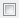Main Content

## Enable run-time recursion for MATLAB functions

### Description

Allow recursive functions in code that is generated for MATLAB® code that contains recursive functions. This parameter applies to MATLAB code in a MATLAB Function block, a Stateflow® chart, or a System object associated with a MATLAB System block. Some coding standards, such as MISRA®, do not allow recursion. To increase the likelihood of generating code that is compliant with MISRA C®, clear this option.

Category: Simulation Target > Advanced parameters

### Settings

Default: OnOn

Enables run-time recursion for code generation of MATLAB code that contains recursive functions.Off

Disables run-time recursion for code generation of MATLAB code that contains recursive functions. If run-time recursion is disabled, and the MATLAB code requires run-time recursion, code generation fails.

### Command-Line Information

 Parameter: `EnableRuntimeRecursion` Value: `'on'` | `'off'` Default: `'on'`

## Support

#### Model-Based Design for Embedded Control Systems

Download white paper